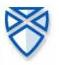# Limitations Of The Sum() Function

10 replies to this topic

### #1fiberoptik

fiberoptik

•• Members
•• 70 posts

• Calculators:
ClassPad 300, FX-850P, FX-7700G, Voyage 200

Posted 27 December 2004 - 02:45 PM

Hello again people, i've had another disappointment with CP.
When i try to calculate sum(1/x^2, x, 1, oo) CP does nothing (returns the expression in symbolic form). I think it can only calculate sums with 200 steps, because if i write sum(1/x^2,x,500,700) it calculates. My disappointment is that i've been playing with a Voyage 200, and it calculates sum(1/x^2,x,1,oo), and also calculates sum(1/x^2,x,1,200) much faster than CP (strange, if CP processor is faster than TI). By the way the result of sum(1/x^2,x,1,oo) is 1.6...(don't remember the rest). It's a Dirichlet serie (sum 1/x^n, if n>1 the serie is convergent).

Is it possible that the new OS has improvements in the CAS engine ?

### #2Overlord

Overlord

Casio Technician

•• Moderator
•• 355 posts
• Gender:Male
• Location:Brussels - Belgium
• Interests:Math Researcher

• Calculators:
My head - C300 OS 3.00 - G100 Rom 1.02 - G65 - G60 - G25

Posted 27 December 2004 - 03:26 PM

(the result of the sum is pi?/6)

do you know how does the v200 calculate the sum (1->inf) ? and what's the result when you sum (1/x,x,1,inf) ?

don't know about the new os...

### #3fiberoptik

fiberoptik

•• Members
•• 70 posts

• Calculators:
ClassPad 300, FX-850P, FX-7700G, Voyage 200

Posted 27 December 2004 - 03:54 PM

Overlord, sum(1/x,x,1,inf) is divergent, don't have result.
Is i said, 1/x^n is a Dirichlet serie, if n>1 the serie is convergent, if n<=1 the serie is divergent. I don't know how the v200 calculate the sum.

fiberoptik

### #4Overlord

Overlord

Casio Technician

•• Moderator
•• 355 posts
• Gender:Male
• Location:Brussels - Belgium
• Interests:Math Researcher

• Calculators:
My head - C300 OS 3.00 - G100 Rom 1.02 - G65 - G60 - G25

Posted 27 December 2004 - 04:20 PM

i know it's divergent (i have analysis exam in 22 days, arghhh), i just wanted to know the calc answer

### #5fiberoptik

fiberoptik

•• Members
•• 70 posts

• Calculators:
ClassPad 300, FX-850P, FX-7700G, Voyage 200

Posted 27 December 2004 - 05:00 PM

I also will have Analysis exam is more or less 20 days...
As for the serie, i suppose the calc answer is impossible or something like it...

### #6fiberoptik

fiberoptik

•• Members
•• 70 posts

• Calculators:
ClassPad 300, FX-850P, FX-7700G, Voyage 200

Posted 28 December 2004 - 07:42 PM

I've tried with Derive (Texas software) and the result of Sum(1/x,x,1,inf) is inf.

Regards

fiberoptik

### #7SoftCalc

SoftCalc

Casio Technician

•• Members
•• 406 posts
• Location:Portland, OR USA

• Calculators:
ClassPad 300 , AFX 2.0, HP-48/49/50, TI-89/92/Voyager, HP Expander, etc...

Posted 28 December 2004 - 10:52 PM

It looks like this has been improved and now works. Now sum(1/x^2, x, 1, oo) --> pi?/6.

Unfortunately I don't know if this improvement was made for the upcoming OS 2.0 or after that. If it was done after then you'll have to wait until the release after 2.0.

### #8fiberoptik

fiberoptik

•• Members
•• 70 posts

• Calculators:
ClassPad 300, FX-850P, FX-7700G, Voyage 200

Posted 30 December 2004 - 02:39 PM

Thanks SoftCalc, i'm very pleased to know that. Does that mean that it can calculate sums with more than 200 steps ?

Best regards

fiberoptik

### #9SoftCalc

SoftCalc

Casio Technician

•• Members
•• 406 posts
• Location:Portland, OR USA

• Calculators:
ClassPad 300 , AFX 2.0, HP-48/49/50, TI-89/92/Voyager, HP Expander, etc...

Posted 31 December 2004 - 07:44 PM

Thanks SoftCalc, i'm very pleased to know that. Does that mean that it can calculate sums with more than 200 steps ?

<{POST_SNAPBACK}>

In infinite sum is different than a sum with a large number of steps. I don't know if the 200 step limit has been changed or not. You can always doe more than 200 steps in a piecewise function.

What type of problem are you trying to solve that uses more than 200 steps? I think the assumption is the CAS returns a symbolic solution so more than 200 steps would normally create a symbolic expression that is way to big. Even if it is a numeric result it can become huge if there are any nested radicals. The only exceptions I can think of are cases where the result doesn't have any radicals or if you are in approx (floating point) mode. Maybe in this case it should solve more than 200 steps if the result will be purely numeric.

### #10fiberoptik

fiberoptik

•• Members
•• 70 posts

• Calculators:
ClassPad 300, FX-850P, FX-7700G, Voyage 200

Posted 03 January 2005 - 02:18 PM

Once again Softcalc, thank you for your answer. The Piecewise function is a good idea in fact.
I suppose that the TI calc use some kind of theoretical result to calculate sums to inf, maybe that's why it could return the result.
In fact i'm not trying to calculate nothing, i was only comparing some results of my Analysis class, and because CP couldn't calculate sums to inf, i tried to decrease the steps. Anyway, even if i don't need it, is good to know it is there.I only tried sum(1/x^2,x,1,n) wich is a numerical result., when i put n=250 or 300, CP returns the symbolic form of sum(1/x^2,x,1,n).

Regards

fiberoptik

### #11Overlord

Overlord

Casio Technician

•• Moderator
•• 355 posts
• Gender:Male
• Location:Brussels - Belgium
• Interests:Math Researcher

• Calculators:
My head - C300 OS 3.00 - G100 Rom 1.02 - G65 - G60 - G25

Posted 03 January 2005 - 08:52 PM

on the cp manager : when i try with n=250 : no problem, it displays the numerical result (in decimal mode)
n=251 no problem
n=256=2^8 no problem
n=257 returns the symbolic form

#### 0 user(s) are reading this topic

0 members, 0 guests, 0 anonymous users